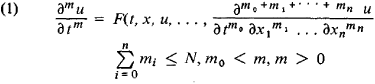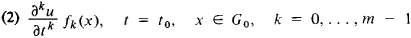# Cauchy Problem

Also found in: Wikipedia.

## Cauchy problem

[kō·shē ‚präb·ləm]
(mathematics)
The problem of determining the solution of a system of partial differential equation of order m from the prescribed values of the solution and of its derivatives of order less than m on a given surface.
McGraw-Hill Dictionary of Scientific & Technical Terms, 6E, Copyright © 2003 by The McGraw-Hill Companies, Inc.
The following article is from The Great Soviet Encyclopedia (1979). It might be outdated or ideologically biased.

## Cauchy Problem

one of the fundamental problems of the theory of differential equations, first studied systematically by A. Cauchy. It consists in finding a solution u(x, t), for x = (xi, …, xn), of a differential equation of the formsatisfying the initial conditionswhere G0—the carrier of the initial data—is a region in the hyperplane t = t0 of the space of variables x1, …, xn. When F and fk, for k = 0, …, m — 1, are analytic functions of their arguments, then the Cauchy problem (1), (2) always has a unique solution in some region G of the space of variables t, x containing G0. This solution, however, can prove to be unstable (that is, a small change in the initial data can cause a large change in the solution), for example, for cases when equation (1) is elliptic. If equation (1) is not hyperbolic and the initial data are not analytic, then the Cauchy problem (1), (2) can lose meaning.

### REFERENCES

Courant, R., and D. Hilbert. Metody matematicheskoi fiziki, vol. 2. Moscow-Leningrad, 1951. (Translated from German.)
Tikhonov, A. N., and A. A. Samarskii. Uraveniia matematicheskoi fiziki, 3rd ed. Moscow, 1966.

References in periodicals archive ?
Among their topics are geometric methods for stochastic dynamical systems, an averaging principle for multi-valued stochastic differential equations driven by G-Brownian motion, Holder estimates for solutions of stochastic nonlocal diffusion equations, the Cauchy problem for a generalized Ostrovsky equation with positive dispersion, the smooth approximation of Levy processes in Skorokhod space, and error estimation on projective integration of expensive multiscale stochastic simulation.
The Cauchy problem for regular equations was investigated, e.g., in well known works [3,4,5,7,9,12], but for singularly perturbed equations it has not been considered previously.
For the local and global well-posedness of the Cauchy problem we refer to [12-14] and references cited therein.
In this paper, we are interested in the Cauchy problem of the Chern-Simons-Schrodinger equations coupled with a neutral field (CSSn) in [R.sup.1+2]:
(13) In the paper titled "New Result on the Critical Exponent for Solution of an Ordinary Fractional Differential Problem," the authors gave some background of fractional Cauchy problems and corrected an existing result of Cauchy problem for the fractional differential inequality, and they also gave an example to illustrate the statement.
In this paper, we initiate the question of the attractivity of solutions for Cauchy problem (1).
In the final section we show some numerical results for an ill-posed Cauchy problem. Without stability of finite difference approximation, it is difficult to observe convergence property on the standard double precision arithmetic environments of computers, but it is possible to do it on a multiple-precision environment (e.g.
It is easy to see that the solution of the homogeneous Cauchy problem
In fact, due to its peculiar formulation, it leads to view the usual Einstein equations as merely initial conditions following the Cauchy problem.
Denoting by S([phi]) the solution set of the Cauchy problem related to (4.2), with x(0) = [x.sub.0], and by S([psi]) the set of all solutions of the Cauchy problem related to (4.3), with x(0) = [x.sub.0], we have S([phi]) = S([psi]).
In the current paper, we consider the following Cauchy problem:
In this paper we utilize the generalized Drazin inverse for closed linear operators to obtain explicit solutions to two types of abstract Cauchy problem. The first type is the heat equation with operator coefficient.

Site: Follow: Share:
Open / Close